#### 期刊菜单

Research on the Development Trend of the Advantageous Industries in the Five Provinces of Southwest China Based on the Location Quotient Index
DOI: 10.12677/SD.2023.131023, PDF, HTML, XML, 下载: 18  浏览: 35  科研立项经费支持

Abstract: Regional coordinated development strategy is an important direction of the current national in-dustrial development, and it is also a current research hotpot. In this paper, the grey prediction model based on location quotient index is applied, combined with the relevant economic data re-sources of the National Bureau of Statistics in Chongqing, Sichuan, Guizhou, Yunnan and Tibet, the advantageous industries of the regional economy in the five southwestern provinces are analyzed in depth. From the perspective of quantitative analysis, the development status and future devel-opment trend of regional advantageous industries are empirically studied, and the regional eco-nomic development of the five southwestern provinces is studied from the industry and regional scale. Finally, on the basis of empirical research, it points out the problems existing in the devel-opment of regional advantageous industries in the five southwestern provinces, and puts forward targeted policy recommendations for the future coordinated development of the five southwestern provinces.

1. 引言

2. 文献回顾

3. 模型建立

3.1. 区位商

3.2. 灰色预测模型

$\frac{\text{d}{x}^{\left(r\right)}\left(t\right)}{\text{d}t}+a{x}^{\left(r\right)}\left(t\right)=b$ (1)

${x}^{\left(r\right)}\left(k\right)+{x}^{\left(r\right)}\left(k-1\right)+a{z}^{\left(r\right)}\left(k\right)=b$ (2)

${\stackrel{^}{x}}^{\left(r\right)}\left(k\right)=\left({x}^{0}\left(1\right)-\frac{b}{a}\right){\text{e}}^{-ak}+\frac{b}{a}$ (3)

$r=1$ ，FGM(1,1)模型退化为经典的GM(1,1)模型。文献  曾指出，GM(1,1)模型的精度极大程度受限于发展系数a的值。为解决这一问题，诸多学者提出过众多解决方案，其中离散灰色模型DGM(1,1)是比较有效的改进方法。类似FGM(1,1)模型，将分数阶引入DGM(1,1)模型，可以得到离散分数阶灰色模型FDGM(1,1)。

4. 实证检验

4.1. 样本选取

4.2. 实证分析

(一) 基于区位商指标的西南五省(市、区)区域产业聚集度分析Table 1. Regional quotient of industries in the five provinces of southwest China in 2021

(二) 基于区位商指标的西南五省(市、区)优势产业动态演进分析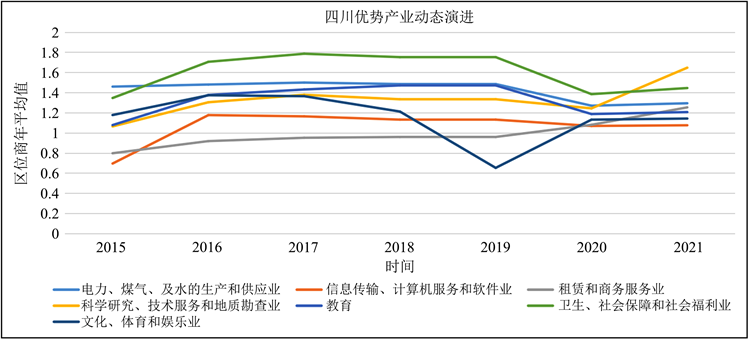Figure 1. Average value of location quotient of all urban areas in Sichuan Province from 2015 to 2021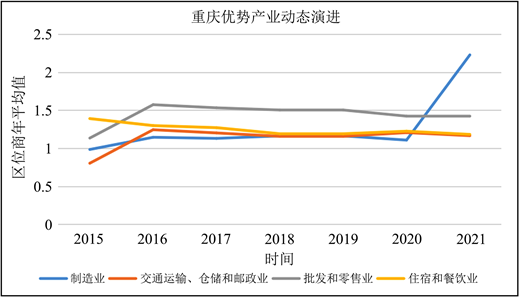Figure 2. Average value of location quotient of all urban areas in Chongqing and Guizhou from 2015 to 2021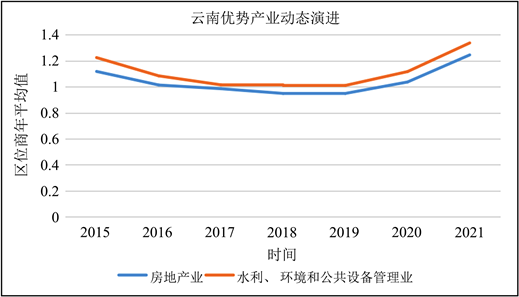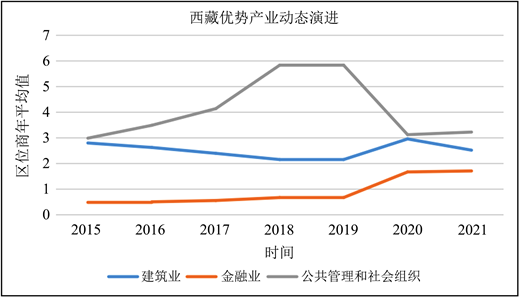Figure 3. Average value of location quotient of all urban areas in Yunnan and Tibet from 2015 to 2021

(三) 基于灰色预测模型的西南五省优势产业发展趋势分析

$\text{MSE}=\frac{1}{N}{\sum }_{t=1}^{N}{\left(f-{\stackrel{^}{f}}_{t}\right)}^{2}$ (4)

$\text{MSE}=\sqrt{\frac{1}{N}{\sum }_{t=1}^{N}{\left(f-{\stackrel{^}{f}}_{t}\right)}^{2}}$ (5)

$\text{MSE}=\frac{1}{N}{\sum }_{t=1}^{N}|\left(f-{\stackrel{^}{f}}_{t}\right)|$ (6)Table 2. Annual average value of location quotient of advantageous industries in the five provinces of southwest ChinaTable 3. The performance of different grey models in predicting the average level (location quotient) of advantageous industries in the five southwest provincesTable 4. Prediction of the future development trend of the average value of regional quotient of the five southwestern provinces based on FDGM (1,1) Mode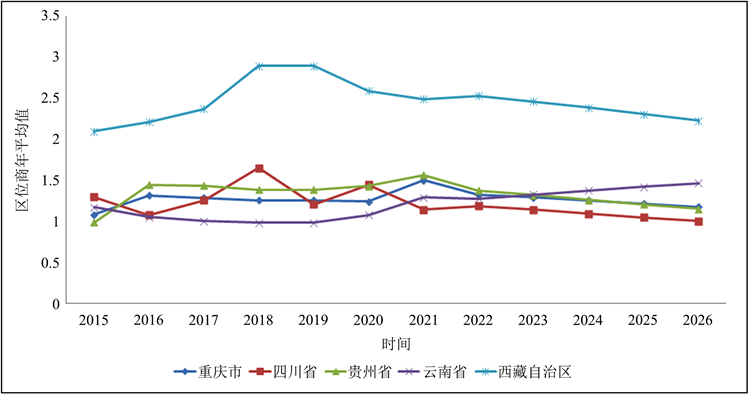Figure 4. Trend of average regional quotient of dominant industries of the five southwest provinces in China

5. 主要结论及政策建议

(一) 建立区域战略联盟，形成层级分工、错位发展的区域格局

(二) 通过区域优势产业动态演进和发展趋势分析，及时调整区域产业政策，促进区域协同发展

(三) 拓展途径，引导聚集

NOTES

*通讯作者。

1数据来源：中国统计年鉴http://www.stats.gov.cn/search/s?qt=%E7%BB%9F%E8%AE%A1%E5%B9%B4%E9%89%B4。

2这里平均值计算标准为，先筛选出区域中区位商最大的一级行业，再计算该区域所有的区位商最大的一级行业区位商平均值(参考表1)。

3https://baijiahao.baidu.com/s?id=1721938126092760078&wfr=spider&for=pc。

4https://www.sc.gov.cn/10462/c102638/jjqjs.shtml。

  Haggett, P. (1977) Locational Models. Edward Amold Ltd., London, 37-69.  Andrew, O. and Mae, D. (2011) An Ecosystem Approach to Recreation Location Quotients. Forests, 2, 993-1012.  Niu, X., Wang, B. and Pekkai, E.K. (2012) Economical Assessment of Forest Ecosystem Services in China: Characteristics and Implications. Ecological Complexity, 11, 1-11. https://doi.org/10.1016/j.ecocom.2012.01.001  陈思, 王宁. 具有区位商法的黑龙江垦区林业优势产业分析[J]. 黑龙江八一农垦大学学报, 2015, 27(3): 106-109.  方鹏程. 区位商视角下的深圳优势产业实证分析[J]. 特区经济, 2011(9): 40-41.  李俊, 兰传海. 基于区位商的区域优势文化产业选择研究——以东北地区为例[J]. 经济问题探索, 2012(5): 41-45.  李军训, 李祖香. 基于区位商的产业集群集聚度研究——以我国纺织产业为例[J]. 南京审计学院学报, 2013(1): 7-13.  唐磊, 曾国平. 区位商分析方法在地区产业比较中的应用——以我国西南和西北地区为对象的实证分析[J]. 经济管理, 2005, 19(4): 55-58.  刘思峰, 邓聚龙. GM(1, 1)模型的适用范围[J]. 系统工程理论与实践, 2000, 20(5): 121-124.  Wu, L.F., Liu, S.F., Yao, L.G., et al. (2013) Grey System Model with the Fractional Order Accumulation. Communications in Nonlinear Science and Numerical Simulation, 18, 1775-1785. https://doi.org/10.1016/j.cnsns.2012.11.017  Xie, N.M. and Wang, R.Z. (2017) A Historic Review of Grey Forecasting Models. Journal of Grey System, 29, 1.  Ma, X., Xie, M., Wu, W.Q., et al. (2019) The Novel Fractional Discrete Multivariate Grey System Model and Its Applications. Applied Mathematical Modelling, 70, 402-424. https://doi.org/10.1016/j.apm.2019.01.039  Zeng, B., Tong, M.Y. and Ma, X. (2020) A New-Structure Grey Verhulst Model: Development and Performance Comparison. Applied Mathe-matical Modelling, 81, 522-537. https://doi.org/10.1016/j.apm.2020.01.014  Wu, W.Q., Ma, X., Zhang, Y.Y., et al. (2020) A Novel Conformable Fractional Non-Homogeneous Grey Model for Forecasting Carbon Dioxide Emis-sions of BRICS Countries. Science of the Total Environment, 707, Article ID: 135447. https://doi.org/10.1016/j.scitotenv.2019.135447  Sen, D., et al. (2021) Forecasting Electricity Consumption of OECD Countries: A Global Machine Learning Modeling Approach. Utilities Policy, 70, Article ID: 101222. https://doi.org/10.1016/j.jup.2021.101222  Fallahpour, A., Wong, K.Y. and Rajoo, S. (2021) An Evolution-ary-Based Predictive Soft Computing Model for Prediction of Electricity Consumption Using Multi Expression Pro-gramming. Journal of Cleaner Production, 283, Article ID: 125187. https://doi.org/10.1016/j.jclepro.2020.125287  Kang, Y.X., Mao, S.H. and Zhang, Y.H. (2021) Variable Order Fractional Grey Model and Its Application. Applied Mathematical Modellling, 97, 619-635. https://doi.org/10.1016/j.apm.2021.03.059  Wu, W.Z., Pang, H.D., Zheng, C.L., et al. (2021) Predictive Analysis of Quarterly Electricity Consumption via a Novel Seasonal Fractional Nonhomogeneous Discrete Grey Model: A Case of Hubei in China. Energy, 229, Article ID: 120714. https://doi.org/10.1016/j.energy.2021.120714  Zeng, B. and Li, H. (2021) Prediction of Coalbed Methane Production in China Based on an Optimized Grey System Model. Energy & Fuels, 35, 4333-4344. https://doi.org/10.1021/acs.energyfuels.0c04195  Gao, M.Y., Yang, H.L., Xiao, Q.Z. and Goh, M. (2022) A Novel Method for Carbon Emission Forecasting Based on Gompertz’s Law and Forecasting Grey Model: Evidence from American Industrial Sector. Renewable Energy, 181, 803-819. https://doi.org/10.1016/j.renene.2021.09.072